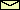# Nonlinear Dynamics

What is "nonlinear dynamics"? Isn't it a ridiculous term like "non-elephant zoology"? To understand this phrase, imagine the time when programmable computers didn't exist. In those days it was impossible to solve a differential equation like the equation of motion of a pendulum driven by a periodic force. That is, the solution couldn't be expressed in terms of well-known functions like in the case of the linearized equation of motion. Nonlinear equations of motion can be solved only in rare cases. For that reason, physicists tried to build their theories on linear differential equations because they are easier to solve. And indeed, the most succesful theories (like electrodynamics and quantum mechanics) are based on linear differential equations. Other even older theories dealing with physical phenomenon closer to everday experience, like fluid dynamics, were less successful because their dynamics is nonlinear.

Yet, the advent of computers in the last decades made it possible to tackle unsolvable nonlinear problems. This possibility led to a completely different view onto dynamical systems and in association with it to a new language about dynamical systems. The basic terms of this language are more geometrically oriented. Instead of quantitative solutions (which can be obtained only numerically in nearly all cases), qualitative aspects are of greater interest like the type of solutions, the stability of solutions, and the bifurcation of new solutions. Nonlinear dynamics became famouse because of the possibility of deterministic chaos, i.e., irregular solutions even though the equation of motion is deterministic. This behavior, that is impossible in linear dynamics, was counterintuitive and therefore attracts much attention not only by mathematicians and physicists, but also by other scientists and even by the general audiance interested in scientific topics. Outside the scientific community, nonlinear dynamics is therefore often called chaos theory, even though not all nonlinear systems behave chaotically.

© 1998 Franz-Josef Elmer,Franz-Josef doht Elmer aht unibas doht ch, last modified Tuesday, July 21, 1998.